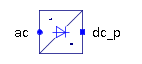Reluctance - MapleSim Help

Fundamental Wave Reluctance

Salient ReluctanceDescription The Fundamental Wave Reluctance (or Reluctance) component models a complex reluctance.Equations $\frac{\mathrm{\pi }}{2}\Re \left({\stackrel{^}{V}}_{m}\right)={\stackrel{^}{R}}_{{m}_{d}}\Re \left(\stackrel{^}{\mathrm{\Phi }}\right)$ $\frac{\mathrm{\pi }}{2}\Im \left({\stackrel{^}{V}}_{m}\right)={\stackrel{^}{R}}_{{m}_{q}}\Im \left(\stackrel{^}{\mathrm{\Phi }}\right)$ ${\stackrel{^}{V}}_{m}={\stackrel{^}{V}}_{{m}_{p}}-{\stackrel{^}{V}}_{{m}_{n}}$ $\stackrel{^}{\mathrm{\Phi }}={\stackrel{^}{\mathrm{\Phi }}}_{p}=-{\stackrel{^}{\mathrm{\Phi }}}_{n}$Variables

 Name Units Description Modelica ID ${\stackrel{^}{V}}_{m}$ $\frac{1}{A}$ Complex magnetic potential difference V_m $\stackrel{^}{\mathrm{\Phi }}$ $\mathrm{Wb}$ Complex magnetic flux PhiConnections

 Name Description Modelica ID ${\mathrm{port}}_{p}$ Positive complex magnetic port port_p ${\mathrm{port}}_{n}$ Negative complex magnetic port port_nParameters

 Name Default Units Description Modelica ID ${\stackrel{^}{R}}_{{m}_{d}}$ ${H}^{-1}$ Reluctance, direct-axis component Rmd ${\stackrel{^}{R}}_{{m}_{q}}$ ${H}^{-1}$ Reluctance, quadrature-axis component RmqModelica Standard Library The component described in this topic is from the Modelica Standard Library. To view the original documentation, which includes author and copyright information, click here.